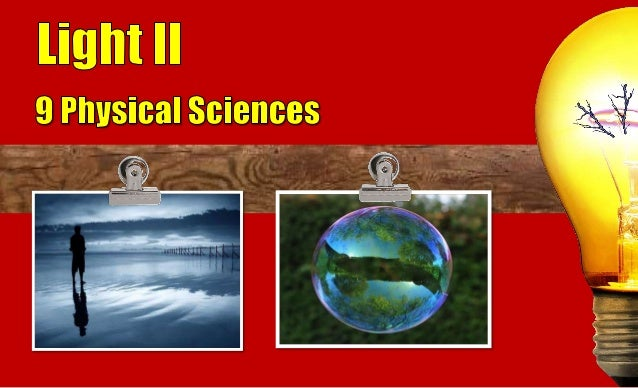Successfully reported this slideshow.Upcoming SlideShare
×

# 9 PS Light II AO

415 views

Published on

This presentation is one of the series as part of the Australian Curriculum for Year 9 Science students. It covers the Physical Sciences (PS) strand of Science Understanding.

9 PS Light II AO covers the theme of 'Energy transfer through different mediums can be explained using wave and particle models (ACSSU182)'.

The elaboration is exploring the properties of waves, and situations where energy is transferred in the form of waves, such as sound and light.

Light II covers the Law of Reflection.

Published in: Education
• Full Name
Comment goes here.

Are you sure you want to Yes No• Be the first to comment

### 9 PS Light II AO

1. 1. The incident ray, the reflected ray and the normal line all line in the same plane. PLANE MIRROR Ray of incidence Ray of reflection Normal line
2. 2. The incident ray or ray of incidence refers to the light ray that is directed towards the plane mirror. PLANE MIRROR Ray of incidence
3. 3. The normal line refers to an imaginary line that is perpendicular to the plane mirror. PLANE MIRROR Normal line
4. 4. The reflection ray or ray of reflection refers to the ray that is directed away from the plane mirror. PLANE MIRROR Ray of reflection
5. 5. PLANE MIRROR Angle of incidence = angle of reflection i° = r° i° r°
6. 6. PLANE MIRROR The angle of incident (i°) is the angle between the normal line and the ray of incidence. i°
7. 7. PLANE MIRROR r° The angle of reflection (r°) is the angle between the normal line and the ray of reflection.
8. 8. Smooth surfaces like the calm water allows light to be reflected evenly. This is known as a specular reflection.
9. 9. Rough surfaces like the rippled and paddled water allows light to be reflected unevenly. This is known as a diffuse reflection.
10. 10. The figure shows a light ray striking a surface of a plane mirror at an angle of 50°. 50° (a) Determine the angle of incidence. (b) Complete the ray of reflection.
11. 11. The figure shows a light ray striking a surface of a plane mirror at an angle of 50°. 50° (a) Determine the angle of incidence. ANSWER The angle of incidence is between the ray of incidence and the normal line. The normal line is perpendicular to the plane mirror. 90° – 50° = 40° 40° Normal line
12. 12. The figure shows a light ray striking a surface of a plane mirror at an angle of 50°. 50° (b) Draw the ray of reflection. 40° 40° ANSWER i = 40° i = r = 40° Ray of reflection is drawn away from the normal.
13. 13. Complete the diagram by sketching reflected rays for an uneven surface.
14. 14. Complete the diagram by sketching reflected rays for an uneven surface. ANSWER The reflected rays of the incident rays are drawn randomly but the ray is still a straight line.
15. 15. Real Object Virtual Image Rays of light do not meet to produce the image.
16. 16. 3. Upright (normal way up). 1. Same size as the object. 2. Virtual.
17. 17. 4. Appears located far inside the mirror as objects are in front. 5. Lateral inversion (reverses left and right).